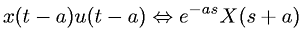Equations > Signal Processing > Laplace Transform Properties > Laplace transform time domain shifting property

### Laplace transform time domain shifting propertyLatex Code:

MathML Code:

 $x\left(t-a\right)u\left(t-a\right)⇔{e}^{-\mathrm{as}}X\left(s+a\right)$

MathType 5.0: